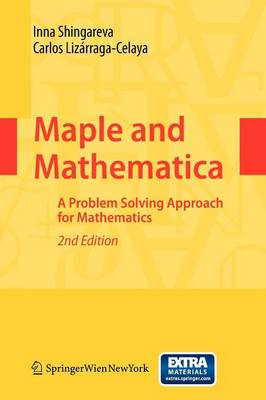•# Maple and Mathematica: A Problem Solving Approach for Mathematics

(author), (author)
£61.99
Mixed media product 484 Pages / Published: 01/09/2009
• We can order this from the publisher

UK delivery within 3-4 weeks

In the history of mathematics there are many situations in which cal- lations were performed incorrectly for important practical applications. Let us look at some examples, the history of computing the number ? began in Egypt and Babylon about 2000 years BC, since then many mathematicians have calculated ? (e. g. , Archimedes, Ptolemy, Vi` ete, etc. ). The ?rst formula for computing decimal digits of ? was disc- ered by J. Machin (in 1706), who was the ?rst to correctly compute 100 digits of ?. Then many people used his method, e. g. , W. Shanks calculated ? with 707 digits (within 15 years), although due to mistakes only the ?rst 527 were correct. For the next examples, we can mention the history of computing the ?ne-structure constant ? (that was ?rst discovered by A. Sommerfeld), and the mathematical tables, exact - lutions, and formulas, published in many mathematical textbooks, were not veri?ed rigorously . These errors could have a large e?ect on results obtained by engineers. But sometimes, the solution of such problems required such techn- ogy that was not available at that time. In modern mathematics there exist computers that can perform various mathematical operations for which humans are incapable. Therefore the computers can be used to verify the results obtained by humans, to discovery new results, to - provetheresultsthatahumancanobtainwithoutanytechnology. With respectto our example of computing?, we can mention that recently (in 2002) Y. Kanada, Y. Ushiro, H. Kuroda, and M.

Publisher: Springer Verlag GmbH
ISBN: 9783211994313
Number of pages: 484
Weight: 765 g
Dimensions: 235 x 155 x 25 mm
Edition: 2nd ed. 2009